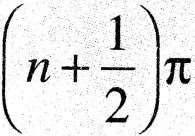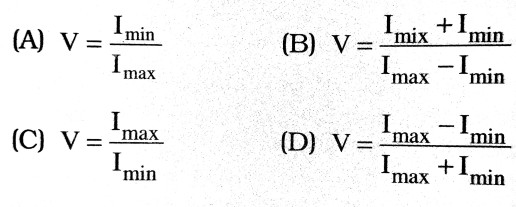1. Poisson’s bright spot was discovered by:

(A) Fresnel
(B) Rayleigh
(C) Poisson
(D) Fraunhoffer

2. The theory of expanding universe is confirmed by the observation of the spectral lines of the star, which shows :

(A) green shift
(B) red shift
(C) violet shift
(D) yellow shift

3. The application of Doppler effect are :

(A) Doppler spectrometer
(C) Doppler velocimeter
(D) All of these

4. Which of the following is conserved when light waves interefere ?

(A) phase
(B) intensity
(C) amplitude
(D) none of these

5. In Young double slit experiment, a minimum is obtained when the phase difference of the superposing waves, is (n ∈ I):

(A) nπ
(B)(C) (2n +1)π
(D) zero

6. The angle of minimum deviation of a prism depends ujpn the aggie of:

(A) incidence
(B) reflection
(C) prism
(D) none of these

7. The minimum value of the refractive index is :

(A) zero
(B) 1
(C) less than 1 but not zero
(D) more than 1

8. Polarization of light prove the :

(A) corpuscular nature of light
(B) quantum nature of light
(C) Transverse wave nature of light
(D) Longitudinal wave nature of light

9. The focal length of the lens is 50 cm; then its power is :

(A) + 2D
(B) + 1
(C) – 2D
(D) – 1D

10. In the above question, the number of the lens is :

(A) + 2
(B) + 1
(C) -2
(D) -1

11. The refractive index of diamond is about :’

(A) 1
(B) 1.42
(C) 2.42
(D) 4.24

12. Two lenses having power +6D and -4D are placed in contact. The power of the combination is

(A) – 2D
(B) – 4D
(C) + 4D
(D) + 2D

13. Diffraction was discovered by :

(A) Grimaldi
(B) Thomas Young
(C) Malus
(D) Huygens

14. The electromagnetic wave theory of light fails to explain :

(A) compton effect
(B) photoelectric effect
(C) neither (A) nor (B)
(D) Both (A) and (B)

15. In an interference pattern produced by two identical slits, the intensity at the site of central maxima is I. The intensity at the same spot when either of the two slit is closed is I0, then

(A) I = I0
(B) I = 2I
(C) I = 4I0
(D) There is no relation between I and I0 .

16. In Y.D.S. Expt. the maximum occurs for sin θ = mλ/d with d =

(A) Distance between slit and screen
(B) Distance between dark and bright fringes
(C) Distance between slits
(D) Width of fringes

17. When viewed in white light, soap bubble show colours because of :

(A) Interference
(B) Scattering
(C) Diffraction
(D) Dispersion

18. The penetration of light into the region of geometrical shadow is called

(A) polarisation
(B) diffraction
(C) refraction
(D) interference

19. Which of the following cannot be polarised ?

(B) Ultra-violet rays
(C) Infrared rays
(D) Ultrasonic waves

20. Electromagnetic waves are transverse in nature. This can be demonstrated by :

(A) reflection
(B) diffraction
(C) polarisation
(D) interference

21. Which of the following is used in optical fibres ?

(A) reflection
(B) scattering
(C) total internal reflection
(D) interference

22. Polaroid glass is used in sun glasses because :

(A) it is cheaper
(B) it is fashionable
(C) it has good colour
(D) it reduces the light intensity to almost half on account of polarisation

23. The visibility V of an interference pattern is :